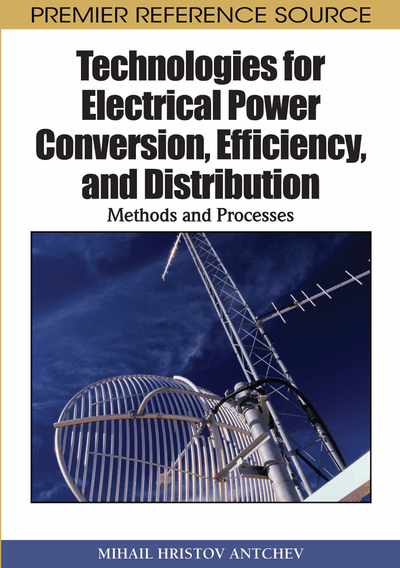# DC/AC Conversion

DOI: 10.4018/978-1-61520-647-6.ch007
Available
\$37.50
No Current Special Offers

## Abstract

The operational principle of converters from DC into AC electrical powers, so-called inverters, is based on the idea studied in Chapter 3. In this chapter, the autonomic or independent inverters are studied, whose operational frequency is determined in general by their control system (Bedford, 1964).
Chapter Preview
Top

## Basic Indicators

The operational principle of converters from DC into AC electrical powers, so-called inverters, is based on the idea studied in Chapter 3. In this chapter, the autonomic or independent inverters are studied, whose operational frequency is determined in general by their control system (Bedford, 1964). These inverters can be separated in the following basic groups:

• 1.

Current-source inverters: Their special feature is a presence of an inductance with a very high value connected in series with the DC source and thus the source become a current generator. As a result, the current through the devices and in the non-branched part of the output circuit have waveforms close to a square one. Voltage across the load is specified by its type and parameters.

• 2.

Voltage-source inverters: Their name comes from the waveform of their output voltage – it has a specific form which depends only on the control algorithm of the power devices of the inverter circuit and on the type of the circuit at the side of the DC source. More often a capacitor with a high value is connected in parallel to the DC source and thereby the source is a voltage generator. Since a voltage with a specific waveform is applied to the inverter output, waveform of the inverter output current depends on type and parameters of the load.

• 3.

Resonant inverters: Their common feature is the use of resonance processes to switch the power devices. Dependent on the power device type and a topology of the power schema, switching at zero current or zero voltage may be realized decreasing the commutation losses and making the use of the resonant inverters in high frequency appropriate. The supplies of the resonant inverters are more often voltage-sources. The inverter load is a part of the resonance circuit, in which resonance processes develop. These processes are developed at the switching of the power devices and thus the DC source voltage appears to be applied to a series oscillating circuit causing the currents through the power devices and the load to have waveforms close sinusoidal.

• 4.

Z-source inverters: Their special feature is a presence of inductances and capacitors connected in Z-circuit in the input circuit at the DC source side. A special control algorithm is used as a result of which the total source voltage applied to the inverter is higher than the voltage of the DC source.

Different power devices have implementation in inverter realizations. The relative part of the devices has been change during the time. Nowadays, thyristors are applied in the current-source inverters and resonant inverters in comparatively high powers. Power IGBTs are the basic devices applied in the voltage-source inverters and Z-source inverters. The IGBTs have also found a wider implementation in resonant inverters. Power MOSFETs are applied in high frequencies in the resonant inverters. The MOSFET implementation is more limited in the voltage-source inverters.

In general, a tendency of expanding the use of the transistors in DC/AC conversion regardless of the inverter applied is imposed. Therefore, the main attention in this chapter is paid to the transistor inverter circuits.

A fundamental indicator of a conversion is efficiency coefficient showing the ratio of the AC power in the load to the DC power consumed from the source:

(7.1) where inare effective values of the k-th harmonics of the load voltage and load current, respectively, and- is displacement angle between them. In this definition, it is assumed that in general both the load voltage and load current are of non-sinusoidal waveform. Applications and loads, which allow this assumption to be made, exist. In other cases, it is important to supply the load with a voltage whose waveform is as closed as possible to the sinusoidal one. Then only a multiplier of k=1 rest in the numerator of (7.1). This is a characteristic mostly of the voltage-source inverters. Therefore, it is usually necessary to separate only the fundamental harmonic from a non-sinusoidal voltage at the inverter output. In this case, the harmonic spectrum of the output inverter voltage is important. The harmonics following the fundamental are preferable to be of a higher possible frequency to simplify their filtering.

## Complete Chapter List

Search this Book:
Reset